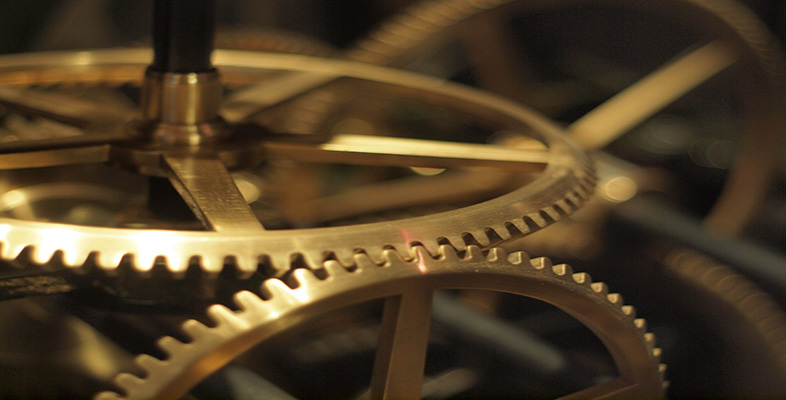Crossing the boundary - analogue universe, digital worlds

This free course is available to start right now. Review the full course description and key learning outcomes and create an account and enrol if you want a free statement of participation.

Free course

4.12 Sound and music

Second only to vision, we rely on sound. Music delights us, noises warn us of impending danger, and communication through speech is at the centre of our human lives. We have countless reasons for wanting computers to reach out and take sounds across the boundary.

Sound is another analogue feature of the world. If you cry out, hit a piano key or drop a plate, then you set particles of air shaking – and any ears in the vicinity will interpret this tremor as sound. At first glance, the problem of capturing something as intangible as a vibration and taking it across the boundary seems even more intractable than capturing images. But we all know it can be done – so how is it done?

The best way into the problem is to consider in a little more detail what sound is. Probably the purest sound you can make is by vibrating a tuning fork. As the prongs of the fork vibrate backwards and forwards, particles of air move in sympathy with them. One way to visualise this movement is to draw a graph of how far an air particle moves backwards and forwards (we call this its displacement) as time passes. The graph (showing a typical wave form) will look like Figure 26.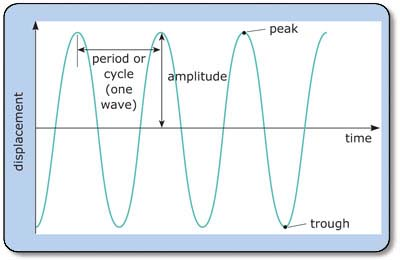Figure 26 Displacement of air particles over time by vibrating a tuning fork

Our particle of air moves backwards and forwards in the direction the sound is travelling. As shown in Figure 26, a cycle represents the time between adjacent peaks (or troughs) and the number of cycles completed in a fixed time (usually a second) is known as the frequency. The amplitude of the wave (i.e. maximum displacement – see Figure 26) determines how loud the sound is, the frequency decides how low or high pitched the note sounds to us. Note, though, that Figure 26 is theoretical; in reality, the amplitude will decrease as the sound fades away.

Of course, a tuning fork is a very simple instrument, and so makes a very pure sound. Real instruments and real noises are much more complicated than this. An instrument like a clarinet would have a complex waveform, perhaps like the graph in Figure 27a, and the dropped plate would be a formless nightmare like Figure 27b.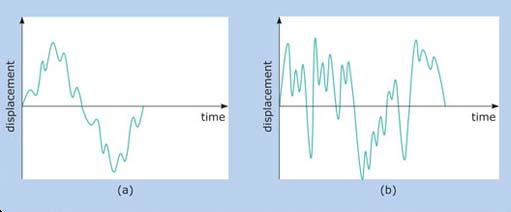Figure 27 Typical waveforms

Exercise 14

Write down a few ideas about how we might go about transforming a waveform into numbers. This is a difficult question, so it might help to think back to the methods we used for encoding images in Subsection 4.3.

Discussion

In a way the answer is similar to the question on how to transform a picture into numbers that I posed in Subsection 4.3. We have to find some way to split up the waveform. We split up images by dividing them into very small spaces (pixels). We can split a sound wave up by dividing it into very small time intervals.

What we can do is record what the sound wave is doing at small time intervals. Taking readings like this at time intervals is called sampling. The number of times per second we take a sample is called the sampling rate.

I'll take the tuning fork example, set an interval of say 0.5 second and look at the state of the wave every 0.5 second, as shown in Figure 28.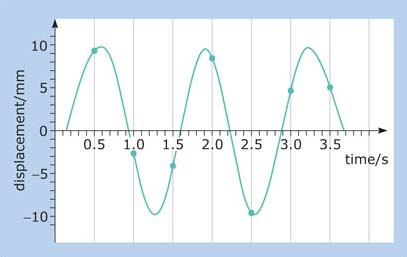Figure 28 Sampling a sound wave

Reading off the amplitude of the wave at every sampling point (marked with dots), gives the following set of numbers:

+9.3, −3.1, −4.1, +8.2, −10.0, +4.0, +4.5

as far as I can judge. Now, if we plot a new graph of the waveform, using just these figures, we get the graph in Figure 29.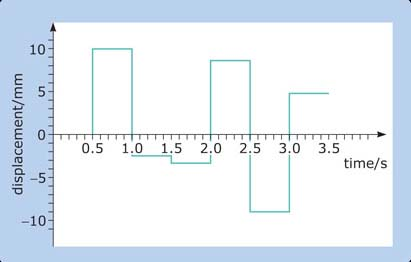Figure 29 Image of a waveform

The plateaux at each sample point represent the intervals between samples, where we have no information, and so assume that nothing happens. It looks pretty hopeless, but we're on the right track.

SAQ 17

How can we improve on Figure 29?

Again, the problem is similar to the one we faced with the bitmapped image. In that case we decreased our spatial division of the image by making the pixel size smaller. In this case we can decrease our temporal splitting up of the waveform, by making the sampling interval smaller.

So, let's decrease the sampling interval by taking a reading of the amplitude every 0.1 second, as in Figure 30.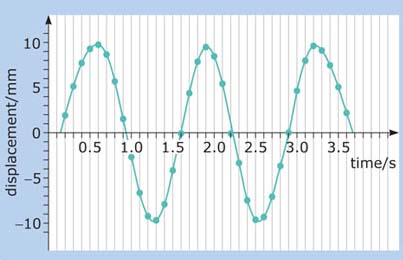Figure 30 Sampling every 0.1 second

Once again, I'll read the amplitude at each sampling point and plot them to a new graph, as in Figure 31, which is already starting to look like the original waveform.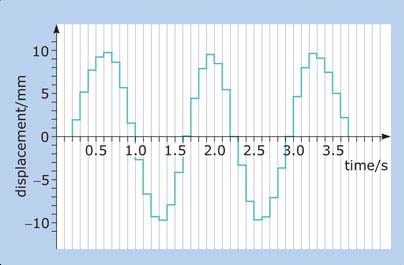Figure 31 Waveform using higher sampling rate

I hope you can see that this process of sampling the waveform has been very similar to the breaking up of a picture into pixels, except that, whereas we split the picture into tiny units of area; we are now breaking the waveform into units of time. In the case of the picture, making our pixels smaller increased the quality of the result, so making the time intervals at which we sample the waveform smaller will bring our encoding closer to the original sound. And just as it is impossible to make a perfect digital coding of an analogue picture, because we will always lose information between the pixels, so we will always lose information between the times we sample a waveform. We can never make a perfect digital representation of an analogue quantity.

SAQ 18

Now we've sampled the waveform, what do we need to do next to encode the image?

Remember that after we had divided an image into pixels, we then mapped each pixel to a number. We need to carry out the same process in the case of the waveform.

This mapping of samples (or pixels) to numbers is known as quantisation. Again, the faithfulness of the digital copy to the analogue original will depend on how large a range of numbers we make available. The human eye is an immensely discriminating instrument; the ear is less so. We are not generally able to detect pitch differences of less than a few hertz (1 hertz (Hz) is a frequency of one cycle per second). So sound wave samples are generally mapped to 16-bit numbers.

M150_1